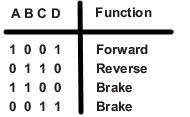# ATmega328P DC motor control with BJT H-bridge

Here program code and circuit diagram for controlling DC motor using BJT based H-bridge are provided. The working principle of the H-bridge used here was explained the earlier post DC Motor with BJT H-bridge motor driver so it will not be explained here.

The following is the circuit diagram of connecting the BJT H-bridge with ATmega328P is shown below.

The four inputs(a,b,c,d) to the H-bridge are connected PB1, PB2, PB3 and PB4 pins of the Atmega328p. Also a switch is also connected PB0 of Atmega328p.

The dc motor is rotated in forward direction when the switch is open and rotated in backward direction when the switch is closed. To do this we need to apply high or low signal at the four inputs a,b,c and d. This pulse sequence is dictated by the following table.So for forward direction a=1, b=0,c=0,d=1 and for backward direction we have a=0,b=1,c=1 and d=0.

Program code

The following is the program code for ATMega328 microcontroller which rotates the dc motor in forward direction if the switch is open and in backward direction if switch is closed.

#include <avr/io.h>

#define a (1<<PB1)
#define b (1<<PB2)
#define c (1<<PB3)
#define d (1<<PB4)
#define sw (1<<PB0)

int main(){
DDRB |= a | b| c |d;
DDRB &= ~sw;
PORTB |= sw;

while (1){
if(PINB & sw){
PORTB |= a;
PORTB &= ~b;
PORTB &= ~c;
PORTB |= d;
}
else if(!(PINB & sw)){
PORTB &= ~a;
PORTB |= b;
PORTB |= c;
PORTB &= ~d;
}
}
return 0;
}


The following video shows animation of how the DC motor control works with BJT based H-bridge motor driver and ATmega328p.

Here we have used h-bridge that uses two NPN transistors 2N3904 and two PNP transistors 2N3906. One can also use all NPN transistors as illustrated in the tutorial Driving DC motor with BJT based H-bridge. Also here we have controlled the DC motor using switch but we can also use atmega328p uart to control the DC motor via PC.